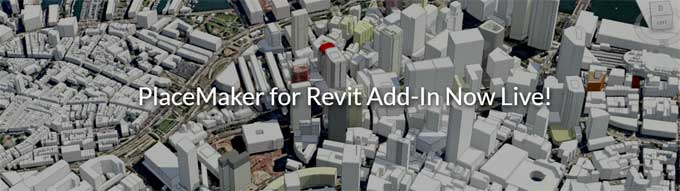# How to Calculate Steel Quantity for Beam, Column and SlabAn important structural element of a building is the slab. There are many different kinds of slabs, but ultimately each one is responsible for moving people from one floor to another in a structure.

A slab can classify as a one way or two-way slab. One-way slabs are divided by main bars running short and distribution bars running long. When the slab length and width are more than 4 meters, two-way slabs typically use, which have bars on both sides.

Proper Calculation the Quantity of Steel for RCC Slab

### 1. Total Number of Main & Distribution Bar

It's first necessary to determine how many bars you will need for the slabs. It is necessary to determine the number of main bars and distribution bars.

• The slab's length is 5 meters = 5000 millimeters.
• The slab has a width of 2 meters = 2000 mm.
• The slab is 0.150 m thick or 150 mm thick.

### Main Bar

• In a slab with a clear cover, the number of bars equals (Total length of slab - 2 times clear cover)/ centre to centre spacing of the bars plus 1.
• Total number of bars = (5000- 2 x 25) 100 plus 1.
• The number of bars is 50.5, which equals 51. The slab requires 51 main bars.

### Distribution Bar

• A distribution bar is equal to (total slab length- 2x clear cover length)/center to center spacing of the bars + 1.
• The distribution bars should be (2000- 2 x 25) /125 + 1.
• Bars of distribution equal 16.6 = 17. The distribution bars number 17 in total.

### 2. Cutting Length of Bar

The slab's clear span is L. Ld is the development length of the bar which is 40 d, where d is its diameter. You can find D by calculating it. A development length is equal to the thickness of the slab minus the clear cover divided by the diameter of the bar.

Calculate the development length by multiplying 150 by 2 x 25 by 12. 88 mm is the development length.

### Main Bar

In order to calculate the cutting length of a Main Bar, use the following formula:

• Length of the main bar to be cut = 2 x Ld + (1 x 0.42 D) ? (2 x 1 d).
• A bar's main cutting length is 2000 + (2 x 40 x 12) + (1 x 0.42 x 88) - (2 x 1 x 12).
• The cutting length is 2972.96 millimeters. 2973 mm are the cutting lengths. 2.973 meters is the cutting length.

### Distribution Bar

Calculating the distribution bar cutting length:

• Distribution bar cutting length equals clear span + 2 x Ld.
• In this example, 5000 plus (2 x 40 x 8) will be the cutting length of the distribution bar.
• 5640 mm is the length of the distribution bar. 5.4 meters is the length of the cutting bar for distribution bars.

### 3. Total Weight of RCC Slab

Quantity Calculation of Main bar Steel

• It requires 51 main bars (as per step 1).
• Each main bar is 2.973 m long (step 2).
• Steel Weight = Total Length x Length of 1 m Steel x Diameter of Steel (D2/ 162).
• Main Bars Total Length = (51 X 2.973).
• The main bars total 151.623m in length.
• Main Bar Weight = Total Length x (D2/ 162).
• The Main Bars weight is 151.623 x (122/162).
• A main bar's weight is 134.776 kilograms.

Quantity Calculation of Distribution bar Steel

• It is necessary to have 17 distribution bars (as outlined in step 1).
• A distribution bar has a length of 5.64 m (as per step 2).
• Distribution bars total length = (17 x 5.64).

• 95.88 m is the total length of the distribution bars.
• Distribution Bar Weight = Total Length x (D2/ 162).
• 95.88 x (102/162) = 37.87 kg are the weight of the Distribution Bars.

### 4. Total Steel Weight for RCC Slab

Sum of weights of main bars and distribution bars for steel for a slab equals the total quantity needed for the slab. 133.77 kg plus 37.87 kg is the total amount of steel required for a slab.

A total of 172.646 kg of steel is required for a slab.

To get more details, watch the following video tutorial.

Video Source: We Civil Engineers